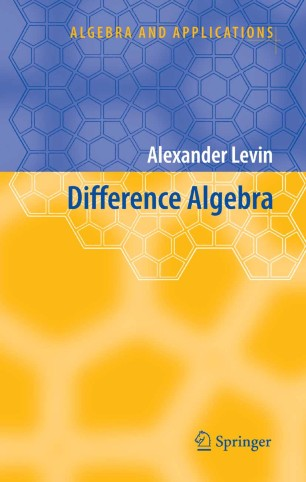# Difference Algebra

• Alexander LevinBook

Part of the Algebra and Applications book series (AA, volume 8)

1. Front Matter
Pages i-xi
2. Pages 1-102
3. Pages 103-154
4. Pages 155-244
5. Pages 245-309
6. Pages 311-370
7. Pages 393-462
8. Pages 463-493
9. Back Matter
Pages 495-519

### Introduction

Difference algebra grew out of the study of algebraic difference equations with coefficients from functional fields in much the same way as the classical algebraic geometry arose from the study of polynomial equations with numerical coefficients. The first stage of the development of the theory is associated with its founder J. F. Ritt (1893 - 1951) and R. Cohn whose book Difference Algebra (1965) remained the only fundamental monograph on the subject for many years. Nowadays, difference algebra has overgrew the frame of the theory of ordinary algebraic difference equations and appears as a rich theory with applications to the study of equations in finite differences, functional equations, differential equations with delay, algebraic structures with operators, group and semigroup rings.

This book reflects the contemporary level of difference algebra; it contains a systematic study of partial difference algebraic structures and their applications, as well as the coverage of the classical theory of ordinary difference rings and field extensions. The monograph is intended for graduate students and researchers in difference and differential algebra, commutative algebra, ring theory, and algebraic geometry. It will be also of interest to researchers in computer algebra, theory of difference equations and equations of mathematical physics. The book is self-contained; it requires no prerequisites other than knowledge of basic algebraic concepts and mathematical maturity of an advanced undergraduate.

### Keywords

Algebra Algebraic difference equation Algebraic structure Difference field extension Difference module Difference polynomial Difference ring difference kernel ring theory

#### Authors and affiliations

• Alexander Levin
• 1
1. 1.The Catholic University of AmericaWashington, D.C.USA